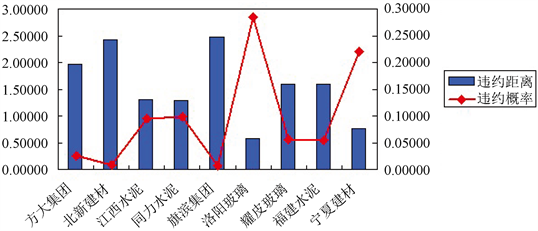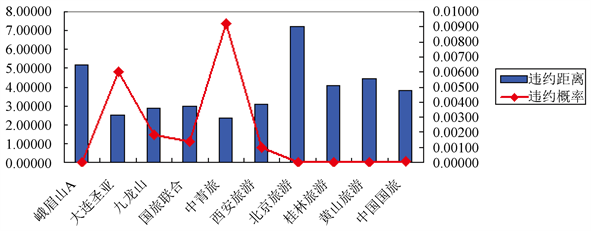基于KMV模型的上市公司债务违约概率度量与实证分析 Measurement and Empirical Analysis of Listed Companies Debt Default Probability via KMV Model

• 全文下载: PDF(1549KB)    PP.6-17   DOI: 10.12677/SA.2019.81002
• 下载量: 152  浏览量: 904   科研立项经费支持

How to measure the default risk of debt of listed companies has always been a hot topic in risk management. In this paper, KMV model is used to analyze relevant data of financial statements issued by listed companies in China, and the probability of their debt default is studied. The default probability of debt of listed companies based on KMV model is established, and the default probability of 41 listed companies in China’s securities market in 2012 is measured by the model. Meanwhile, 41 listed companies were divided into industries for similar analysis and inter-class analysis. The empirical results show that when the company’s asset-liability ratio is higher than 80%, regardless of its net asset liability ratio, it has a high credit risk, while when the company’s asset-liability ratio is lower than 50%, as long as there is no negative return on equity, the company is less likely to default.

1. 研究背景和意义

2. KMV模型建立

KMV公司的KMV模型认为，当企业的资产低于全部债务价值的某个临界平时，企业一般会选择违约，并将这一临界点成为违约点(DPT)。它等于企业短期债务(STD)和一半长期债务(LTD)之和  ：

$DPT=STD+\frac{\text{1}}{\text{2}}LTD$ (1)

$Q=prob\left[{V}_{t}\le DP{T}_{t}\right]$ (2)

$\frac{\text{d}{V}_{t}}{{V}_{t}}=\mu \text{d}t+\sigma \text{d}z$ (3)

${V}_{t}={V}_{0}\mathrm{exp}\left\{\left[\mu -\frac{{\sigma }^{2}}{2}\right]t+\sigma \sqrt{t}{Z}_{t}\right\}$ (4)

$\begin{array}{c}Q=prob\left[\mathrm{ln}{V}_{0}+\left(r-\frac{{\sigma }^{2}}{2}\right)t+\sigma \sqrt{t}{Z}_{t}\le \mathrm{ln}DP{T}_{t}\right]\\ =prob\left[{Z}_{t}\le -\frac{\mathrm{ln}\frac{{V}_{0}}{DP{T}_{t}}+\left(r-\frac{{\sigma }^{2}}{2}\right)t}{\sigma \sqrt{t}}\right]=N\left(-{d}^{*}\right)\end{array}$ (5)

KMV将 ${d}^{\text{*}}$ 定义为违约距离(DD)，即资产价值最终分布的均值与违约临界值之间的距离是资产未来汇报变准成的倍数。

3. 参数估计

3.1. 公司资产市场价值的设定

${V}_{A}=D+{V}_{s}$ (6)

3.2. 违约点

3.3. 上市公司资产市场价值的波动率

${u}_{i}=\mathrm{ln}{s}_{i}-\mathrm{ln}{s}_{i-1}$ ，其中， $i=1,2,3,\cdots ,n$ (7)

$s=\sqrt{\frac{1}{n-1}\underset{i=1}{\overset{n}{\sum }}{\left({u}_{i}-\stackrel{¯}{u}\right)}^{2}}$$s=\sqrt{\frac{1}{n-1}\underset{i=1}{\overset{n}{\sum }}{u}_{i}^{2}-\frac{1}{n\left(n-1\right)}{\left(\underset{i=1}{\overset{n}{\sum }}{u}_{i}\right)}^{2}}$ (8)

3.4. 无风险利率

4. 实证分析

4.1. 样本选取Table 1. Industry distribution of sample companies

4.2. 违约距离和违约概率的计算Table 2. Assets market value and volatility of sample companies

Table 3. Critical point of sample companiesTable 4. Default distance and default probability

4.3. 同类对比

(一) 建材类Figure 1. Building materials categoryTable 5. Financial status of building materials companies

(二) 石油类Figure 2. Category of petroleumTable 6. Financial status of oil companies

(三) 旅游类Figure 3. Tourism categoryTable 7. Financial status of tourism companies

(四) 房地产类Figure 4. Real estate categoryTable 8. Financial status of real estate companies

(五) 同类对比小结

4.4. 类间对比Table 9. Comparison results between categories

5. 结论

NOTES

*通讯作者。

  都红雯, 杨威. 我国对KMV模型实证研究中存在的若干问题及对策思考[J]. 国际金融研究, 2004(11): 22-27.  程鹏, 吴冲锋. 上市公司信用状况分析新方法[J]. 系统工程理论方法应用, 2002(2): 89-93.  郑茂. 基于EDF模型的上市公司信用风险实证研究[J]. 管理工程学报, 2005(3): 151-154.  梁世栋, 李勇, 方兆本. 信用风险模型比较分析[J]. 中国管理科学, 2002(2): 35-43.  程鹏, 吴冲锋, 李为冰. 信用风险度量和管理方法研究[J]. 管理工程学报, 2002(1): 77-73.  Chang, C. (2012) Default Probability of a Captive Credit Bank with Government Capital Injections: A Capped Barrier Option Approach. Economic Modelling, 29, 2444-2450. https://doi.org/10.1016/j.econmod.2012.07.009  Perko, I. (2017) Behaviour-Based Short-Term Invoice Probability of Default Evaluation. European Journal of Operational Research, 257, 1045-1054. https://doi.org/10.1016/j.ejor.2016.08.039  Denzler, S.M., et al. (2006) From Default Probabilities to Credit Spreads: Credit Risk Models Do Explain Market Prices. Finance Research Letters, 3, 79-95. https://doi.org/10.1016/j.frl.2006.01.004  Hainaut, D. and Deelstra, G. (2014) Default Probabilities of a Holding Company, with Complete and Partial Information. Journal of Computational and Applied Mathematics, 271, 380-400. https://doi.org/10.1016/j.cam.2014.04.003  Melgarejo, M. (2018) Beating Market Expectations and the Pricing of Firms’ Probability of Default. Journal of Contemporary Accounting & Economics, 14, 41-51. https://doi.org/10.1016/j.jcae.2018.02.005  Tabak, B.M., Luduvice, A.V.D. and Cajueiro, D.O. (2011) Mod-eling Default Probabilities: The Case of Brazil. Journal of International Financial Markets, Institutions and Money, 21, 513-534. https://doi.org/10.1016/j.intfin.2011.01.007  Cantia, C. and Tunaru, R. (2017) A Factor Model for Joint Default Probabilities. Pricing of CDS, Index Swaps and index Tranches. Insurance: Mathematics and Economics, 72, 21-35. https://doi.org/10.1016/j.insmatheco.2016.10.004  Hao, X., Li, X. and Shimizu, Y. (2013) Finite-Time Survival Probability and Credit Default Swaps Pricing under Geometric Lévy Markets. Insurance: Mathematics and Economics, 53, 14-23. https://doi.org/10.1016/j.insmatheco.2013.04.003  Câmara, A., Popova, I. and Simkins, B. (2012) A Com-parative Study of the Probability of Default for Global Financial Firms. Journal of Banking & Finance, 36, 717-732. https://doi.org/10.1016/j.jbankfin.2011.02.019  Trussel, J. (1997) Default Probability on Corporate Bonds: A Contingent Claims Model. Review of Financial Economics, 6, 199-209. https://doi.org/10.1016/S1058-3300(97)90006-9  Grammenos, C.T., Nomikos, N.K. and Papapostolou, N.C. (2008) Estimating the Probability of Default for Shipping High Yield bond Issues. Transportation Research Part E: Logistics and Transportation Review, 44, 1123-1138. https://doi.org/10.1016/j.tre.2007.10.005  梁琪. 我国商业银行信贷风险管理体系构建探索[J]. 南开经济研究, 2002(6): 14-16.